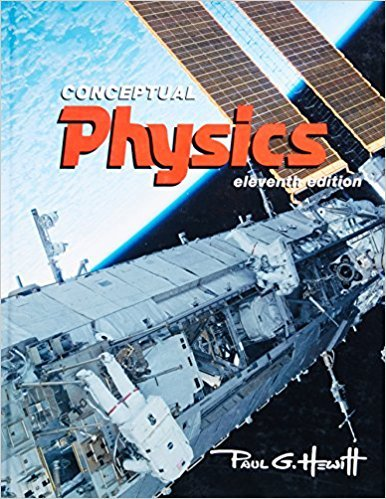×
×

# Answer: What is the ideal efficiency of an automobileISBN: 9780321568090 120

## Solution for problem 1P Chapter 18

Conceptual Physics | 11th Edition

• Textbook Solutions
• 2901 Step-by-step solutions solved by professors and subject experts
• Get 24/7 help from StudySoup virtual teaching assistantsConceptual Physics | 11th Edition

4 5 0 376 Reviews
15
4
Problem 1P

What is the ideal efficiency of an automobile engine in which fuel is heated to 2700 K and the outdoor air is 270 K?

Step-by-Step Solution:
Step 1 of 3

Temperature of fuel is heated,=2700 K

Temperature  of outdoor air,=270 K

Now we have to find the efficiency of ideal heat engine,

We have equation=1-

Step 2 of 3

Step 3 of 3

##### ISBN: 9780321568090

Conceptual Physics was written by and is associated to the ISBN: 9780321568090. The full step-by-step solution to problem: 1P from chapter: 18 was answered by , our top Physics solution expert on 11/08/17, 04:03AM. This textbook survival guide was created for the textbook: Conceptual Physics, edition: 11. The answer to “What is the ideal efficiency of an automobile engine in which fuel is heated to 2700 K and the outdoor air is 270 K?” is broken down into a number of easy to follow steps, and 24 words. Since the solution to 1P from 18 chapter was answered, more than 223 students have viewed the full step-by-step answer. This full solution covers the following key subjects: air, automobile, efficiency, engine, Fuel. This expansive textbook survival guide covers 19 chapters, and 1312 solutions.

Unlock Textbook Solution